# 最长公共子串、最长公共子序列的Java实现与NLP应用

## 最长公共子串（Longest Common Substring）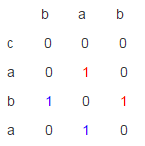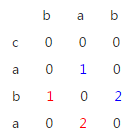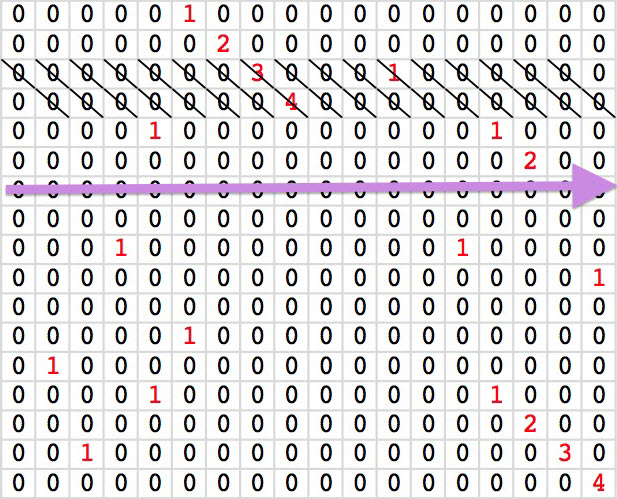A=www.hankcs.com

B=hankcs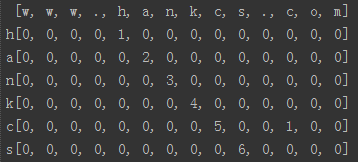``` [w, w, w, ., h, a, n, k, c, s, ., c, o, m]
h[0, 0, 0, 0, 1, 0, 0, 0, 0, 0, 0, 0, 0, 0]
a[0, 0, 0, 0, 0, 2, 0, 0, 0, 0, 0, 0, 0, 0]
n[0, 0, 0, 0, 0, 0, 3, 0, 0, 0, 0, 0, 0, 0]
k[0, 0, 0, 0, 0, 0, 0, 4, 0, 0, 0, 0, 0, 0]
c[0, 0, 0, 0, 0, 0, 0, 0, 5, 0, 0, 1, 0, 0]
s[0, 0, 0, 0, 0, 0, 0, 0, 0, 6, 0, 0, 0, 0]```

### 还可以继续优化吗？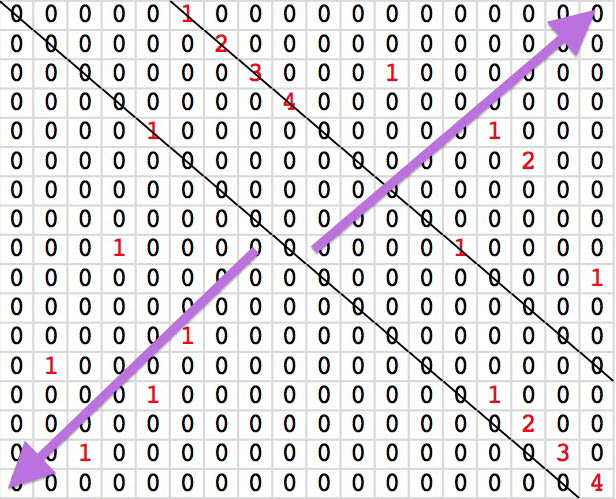### Java代码

```/**
* 求最长公共子串的长度
*
* @author hankcs
*/
public class LongestCommonSubstring
{
public static int compute(char[] str1, char[] str2)
{
int size1 = str1.length;
int size2 = str2.length;
if (size1 == 0 || size2 == 0) return 0;

// the start position of substring in original string
int start1 = -1;
int start2 = -1;
// the longest length of common substring
int longest = 0;

// record how many comparisons the solution did;
// it can be used to know which algorithm is better
int comparisons = 0;

for (int i = 0; i < size1; ++i)
{
int m = i;
int n = 0;
int length = 0;
while (m < size1 && n < size2)
{
++comparisons;
if (str1[m] != str2[n])
{
length = 0;
}
else
{
++length;
if (longest < length)
{
longest = length;
start1 = m - longest + 1;
start2 = n - longest + 1;
}
}

++m;
++n;
}
}

// shift string2 to find the longest common substring
for (int j = 1; j < size2; ++j)
{
int m = 0;
int n = j;
int length = 0;
while (m < size1 && n < size2)
{
++comparisons;
if (str1[m] != str2[n])
{
length = 0;
}
else
{
++length;
if (longest < length)
{
longest = length;
start1 = m - longest + 1;
start2 = n - longest + 1;
}
}

++m;
++n;
}
}
System.out.printf("from %d of str1 and %d of str2, compared for %d times\n", start1, start2, comparisons);
return longest;
}

public static int longestCommonSubstring(String str1, String str2)
{
return compute(str1.toCharArray(), str2.toCharArray());
}
}```

### 测试

```package com.hankcs.test.algorithm;

import com.hankcs.hanlp.algoritm.LongestCommonSubstring;
import junit.framework.TestCase;

public class LongestCommonSubstringTest extends TestCase
{
String a = "www.hankcs.com";
String b = "hankcs";
public void testCompute() throws Exception
{
System.out.println(LongestCommonSubstring.compute(a.toCharArray(), b.toCharArray()));
assertEquals(6, LongestCommonSubstring.compute(a.toCharArray(), b.toCharArray()));
}

public void testLongestCommonSubstring() throws Exception
{
System.out.println(LongestCommonSubstring.longestCommonSubstring(a, b));
assertEquals(6, LongestCommonSubstring.longestCommonSubstring(a, b));
}
}```

### 输出

```from 4 of www.hankcs.com and 0 of hankcs, compared for 84 times
6```

## 最长公共子序列（Longest Common Substring）

```           0                            (如果i=0且j=0)
dp[i][j] = dp[i-1][j-1] + 1            （如果i和j>0，且A[i]=B[j]）
max(dp[i][j-1], dp[i-1][j]) （如果i和j>0，且A[i]!=B[j]）```

### Java代码

```package com.hankcs.hanlp.algoritm;

/**
* @author hankcs
*/
public class LongestCommonSubsequence
{
public static int compute(char[] str1, char[] str2)
{
int substringLength1 = str1.length;
int substringLength2 = str2.length;

// 构造二维数组记录子问题A[i]和B[j]的LCS的长度
int[][] opt = new int[substringLength1 + 1][substringLength2 + 1];

// 从后向前，动态规划计算所有子问题。也可从前到后。
for (int i = substringLength1 - 1; i >= 0; i--)
{
for (int j = substringLength2 - 1; j >= 0; j--)
{
if (str1[i] == str2[j])
opt[i][j] = opt[i + 1][j + 1] + 1;// 状态转移方程
else
opt[i][j] = Math.max(opt[i + 1][j], opt[i][j + 1]);// 状态转移方程
}
}
System.out.println("substring1:" + new String(str1));
System.out.println("substring2:" + new String(str2));
System.out.print("LCS:");

int i = 0, j = 0;
while (i < substringLength1 && j < substringLength2)
{
if (str1[i] == str2[j])
{
System.out.print(str1[i]);
i++;
j++;
}
else if (opt[i + 1][j] >= opt[i][j + 1])
i++;
else
j++;
}
System.out.println();
return opt;
}

public static int compute(String str1, String str2)
{
return compute(str1.toCharArray(), str2.toCharArray());
}
}```

### 测试

```public class LongestCommonSubsequenceTest extends TestCase
{
String a = "Tom Hanks";
String b = "Hankcs";
public void testCompute() throws Exception
{
System.out.println(LongestCommonSubsequence.compute(a, b));
}
}```

### 输出

```substring1:Tom Hanks
substring2:Hankcs
LCS:Hanks
5```

## NLP应用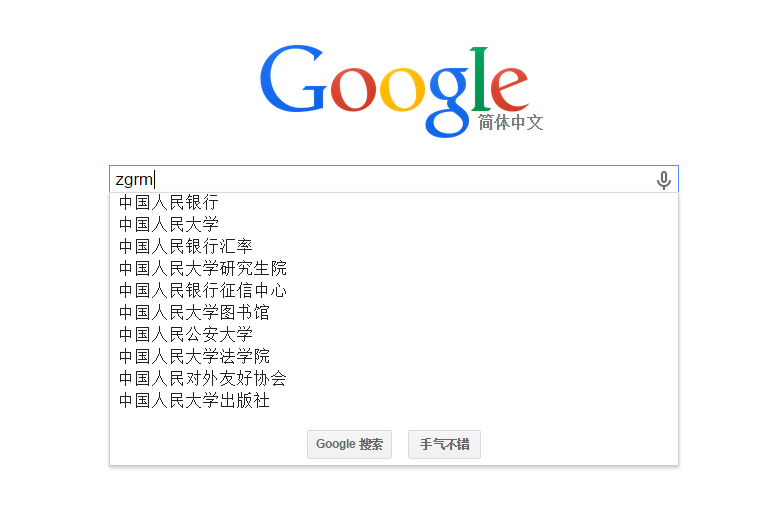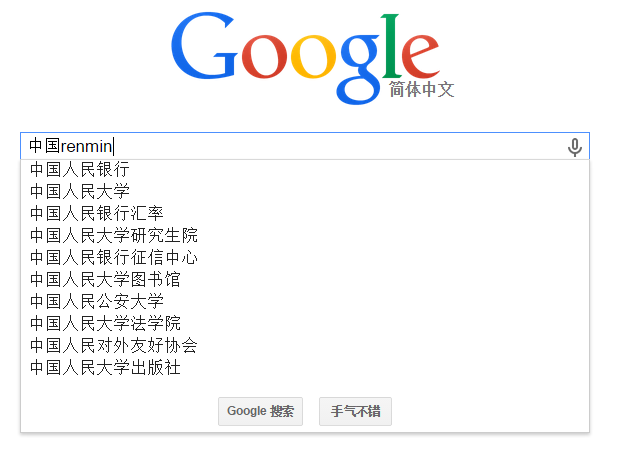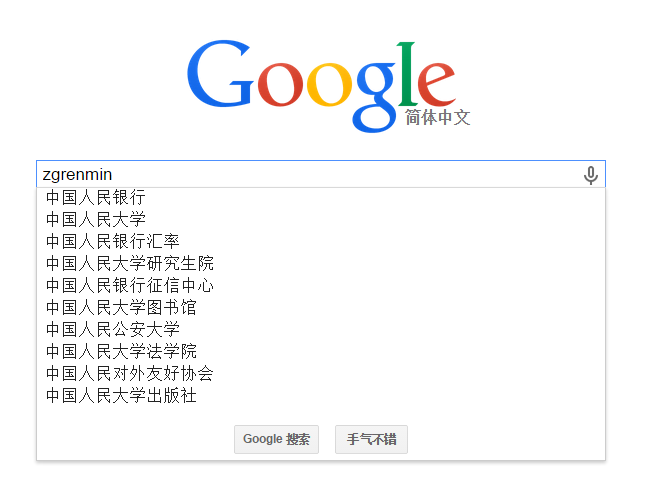```中国人民 [中国人民, 中国人民共和国, 中国人民武装警察部队上海市, 中国人民解放军中华人民共和国, 中国人民解放军热爱, 中国软件, 全国人民, 养护工人们, 最高荣誉, 体会工人们] 180ms
zhongguorenmin [中国人民, 中国人民共和国, 中国人民武装警察部队上海市, 中国人民解放军中华人民共和国, 中国人民解放军热爱, 中国软件, 全国人民, 养护工人们, 最高荣誉, 体会工人们] 66ms
zgrm [中国人民共和国, 中国人民解放军中华人民共和国, 中国人民武装警察部队上海市, 中国人民解放军热爱, 中国人民, 组织个人, 最高荣誉, 副主任张光人, 体会工人们, 个人梦想] 22ms
zgrenmin [中国人民, 全国人民, 中国人民解放军热爱, 中国人民武装警察部队上海市, 中国人民共和国, 中国人民解放军中华人民共和国, 静安人民, 全市人民, 发展人民, 常德人民] 35ms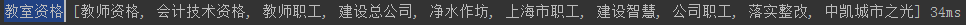## Reference

http://my.oschina.net/leejun2005/blog/117167

http://www.cnblogs.com/ider/p/longest-common-substring-problem-optimization.html

### 评论 1

1. #1

如果早点看到群主的这篇就好了，我的应用场景是OCR识别后，去做精确查询。用到的是lucene的布尔评分后的排序内容后，取结果。做二次排重。排重的标准就是最长公共子串（Longest Common Substring）+编辑距离。不是直接的加是用个比例，70%+30%类似这样。经过测试已经在产品上能用啦。虽然应用不一样。不过看到这个后，原来不同组合的算法可以有不同用途。不过我不会写算法啦，上面的两个算法都是COPY的哈哈，跟博主不是一个级别。

侠盗小明9年前 (2015-04-07)回复